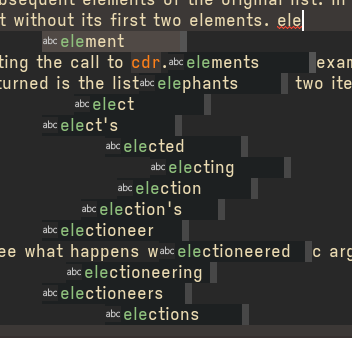# 如何设置 company-mode 的 auto completion 候选词的字体为 fixed-pitch？

Hello，最近想把 Org mode 正文、标题的字体设置成 variable-pitch，更多一点『文档』的感觉， 而让 code、table 等部分还是保持 fixed-pitch。1 个赞

``````(defvar my-fixed-font "Victor Mono"
"Fixed pitch font.")

(defvar my-variable-font "Sarasa Mono SC"
"Variable pitch font.")

(defun my-org-font-setup ()
"Setup variable-pitch fonts for `org-mode'."
(interactive)
(variable-pitch-mode)
(let ((variable-pitch `(:font ,my-fixed-font))
(fixed `(:font ,my-variable-font)))
(custom-theme-set-faces
'user
`(org-level-1 ((t (,@variable-pitch :height 1.5))))
`(org-level-2 ((t (,@variable-pitch :height 1.4))))
`(org-level-3 ((t (,@variable-pitch :height 1.3))))
`(org-level-4 ((t (,@variable-pitch :height 1.2))))
`(org-table ((t (,@fixed))))
`(org-verbatim ((t (,@fixed))))
`(org-formula ((t (,@fixed))))
`(org-code ((t (,@fixed))))
`(org-block ((t (,@fixed)))))))